## Eigenspaces

Let A be an n x n matrix and consider the set E = { xε R n : A x = λ x}. If x ε E, then so is t x for any scalar t, since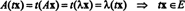Furthermore, if x 1 and x 2 are in E, thenThese calculations show that E is closed under scalar multiplication and vector addition, so E is a subspace of R n . Clearly, the zero vector belongs to E; but more notably, the nonzero elements in E are precisely the eigenvectors of A corresponding to the eigenvalue λ. When the zero vector is adjoined to the collection of eigenvectors corresponding to a particular eigenvalue, the resulting collection,forms a vector space called the eigenspace of A correspondign to the eigenvalue λ. Since it depends on both A and the selection of one of its eigenvalues, the notation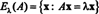will be used to denote this space. Since the equation A x = λ x is equivalent to ( A − λ I) x = 0, the eigenspace E λ( A) can also be characterized as the nullspace of A − λ I: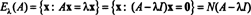This observation provides an immediate proof that E λ( A) is a subspace of R n .

Consider the matrix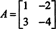The determination of the eigenvectors of A shows that its eigenspaces are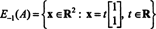and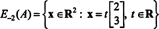E −1( A) is the line in R 2 through the origin and the point (1, 1), and E −2( A) is the line through the origin and the point (2, 3). Both of these eigenspaces are 1‐dimensional subspaces of R 2.

Example 1: Determine the eigenspaces of the matrix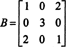First, form the matrix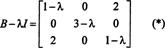The determinant will be computed by performing a Laplace expansion along the second row:The roots of the characteristic equation,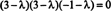are clearly λ = −1 and 3, with 3 being a double root; these are the eigenvalues of B. The associated eigenvectors can now be found. Substituting λ = −1 into the matrix B − λ I in (*) giveswhich is the coefficient matrix for the equation ( B − λ I) x = 0 with λ = −1, which determines the eigenvectors corresponding to the eigenvalue λ = −1. These eigenvectors are the nonzero solutions ofThe identical first and third equations imply that x 1 + x 3 = 0— that is, x 3 = − x 1—and the second equation says x 2 = 0. Therefore, the eigenvectors of B associated with the eigenvalue λ = −1 are all vectors of the form ( x 1, 0, − x 1) T = x 1(1, 0, −1) T for x 1 ≠ 0. Removing the restriction that the scalar multiple be nonzero includes the zero vector and gives the full eigenspace: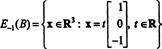Now, sincethe eigenvectors corresponding to the eigenvalue λ = 3 are the nonzero solutions of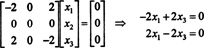These equations imply that x 3 = x 1, and since there is no restriction on x 2, this component is arbitrary. Therefore, the eigenvectors of B associated with λ = 3 are all nonzero vectors of the form (x 1,x 2,x 1) T = x 1(1,0,1) T + x 2(0,1,0) T The inclusion of the zero vector gives the eigenspace: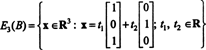Note that dim E −1( B) = 1 and dim E 3( B) = 2.